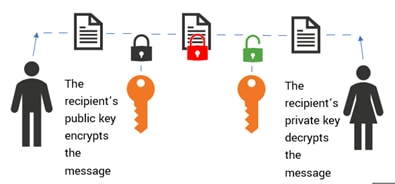LEARN

# Advanced Encryption Standard & AES Rijndael Explained

Advanced Encryption Standard (AES) is a standardization for block cipher published by NIST, the National Institute of Standards and Technology, in 1997.

Block cipher can be seen as a machine or set of instructions that are applied to a fixed length of bits in order to encrypt the data. The algorithms that use the block cipher mechanism are developed under the AES specifications, which replaced the previous Data Encryption Standard (DES).

Keep reading to learn all about the AES standard. Specifically, I’ll cover the popular Rijndael algorithm that won the NIST competition as the chosen AES standard algorithm to ensure data confidentiality## AES Rijndael: How does it work?

Let’s start with a brief description of the Rijndael algorithm (pronounced "rain-doll"). This is a Symmetric Key Encryption scheme developed by two Belgian scientists, Vincent Rijmen and Joan Daemen — hence the name, Rijndael Algorithm.

Symmetric encryption refers to the cryptographic algorithm that uses the same (private) key for encryption and decryption. The decryption function is simply the inverse of the encryption function on the data using the same key. This is an efficient cryptographic method that can be used to secure large amounts of data. According to the AES standard, the key length can be 128, 192 or 256 bits for a block cipher of length 128 bits.This is different from asymmetric encryption, which requires an additional public key verifiable by a certifying authority. Asymmetric encryption is a slower cryptographic process that consumes more resources in comparison — but it provides authenticity and non-repudiation in addition to data confidentiality.

The typical key length size is 2048 bits or higher. Common algorithms used for Asymmetric encryption include:

• Diffie-Helman
• El Gamal
• RSA

(Read cryptography & data encryption intros for more on these concepts.)

## The Substitution-Permutation Network block

Now let’s look at the block cipher that is used to implement the Rijndael AES algorithm. The Substitution-Permutation (SP) Network block performs the instructions as specified by the Rijndael algorithm. S is the substitution block, P is the permutation block.

The SP-network takes a plaintext and encryption keys as the input and returns the encrypted ciphertext. The blocks apply several rounds of alternating layers containing Substitution and Permutation operations. The decryption process is simply the reverse operation of these rounds. This process is described in the following steps:

1. Initial Steps. A fixed length key is generated according to the AES key schedule. A bitwise XOR operation is computed between every data byte (state) and the key.
2. SubBytes. A non-linear transformation of each byte using a lookup table. The data state array is replaced by the data in the substitution box of the SP-network.
3. ShiftRows. The state matrix is transposed — cyclic row transformation by a specified offset. For instance, each element of the second row is shifted by one, third row by two and the 4th row by three states towards the left. This results in the cyclic transposition of the state matrix.
4. MixColumns. Modular multiplication between the state column and the polynomial whose coefficients belong to the finite field. The multiplication and addition of entries in these columns results in the diffusion of the cipher. Diffusion property states that by transforming a single bit in the plaintext — around half of the ciphertext bits should change as a consequence.
5. AddRoundKey. This operation derives a subkey from the original key using the algorithm’s key schedule and adds it to the state bytes using a bitwise XOR operation.

## AES Rijndael security properties

The goal of the AES call for algorithms twofold: To find a cryptographic algorithm candidate that performs better than the preceding DES algorithms and to demonstrate the following security properties for data confidentiality:

• K-Secure. A relative security measure, which ascertains that the cipher does not demonstrate any of the following weaknesses: vulnerable to key-recovery faster than exhaustive key search; symmetry properties in the mapping; ineligible and weak key classes; or related key attacks.
• Hermetic. A block cipher algorithm has this property when it does not demonstrate any security weakness present in most block ciphers of the same key length specification and block type.In addition to demonstrating the K-Secure and Hermetic security properties, the Rijndael algorithm offers the following key advantages.

### Implementation

The algorithm can be implemented on low-memory devices such as smart cards. Speed improvements can be achieved by using smaller state tables for applications facing lower security requirements. The round transformation operation is parallel by design and the block cipher has no bias toward specific processor architectures.

### Design simplicity

The algorithm bases its security on well understood mathematical operations across iterative rounds, which means that it does not rely on obscurity or complex processes to guarantee security.

The algorithm can use variable block and key lengths, depending on the evolving security requirements.

## Understanding the limits

One of the important limitations of this block cipher relates to the decryption process and how it may be implemented in different settings:

• For software implementation, the inverse operation requires different code and tables.
• For hardware, only part of the encryption block circuit can be reused.

## What is Splunk?

This posting does not necessarily represent Splunk's position, strategies or opinion.Posted by